### QUOTIENT Function Examples in Excel, VBA, & Google Sheets

This Tutorial demonstrates how to use the Excel QUOTIENT Function in Excel to calculate the integer portion of division.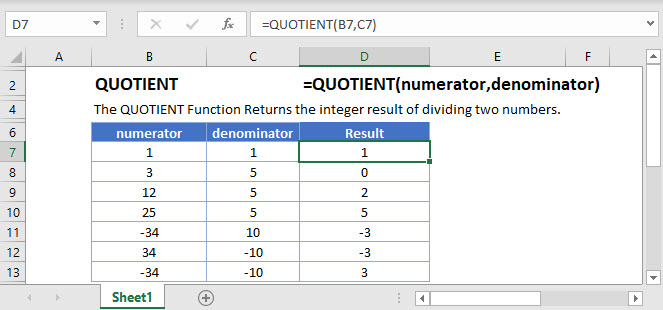## QUOTIENT Function Overview

The QUOTIENT Function Returns the integer result of dividing two numbers.

To use the QUOTIENT  Excel Worksheet Function, select a cell and type: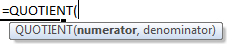(Notice how the formula inputs appear)

## QUOTIENT Function Syntax and Inputs:

numerator – A number for the numerator.

denominator – A number for the denominator.

AutoMacro - VBA Code Generator

## QUOTIENT Function

The QUOTIENT Function divides the number entered and returns the integer portion of their division.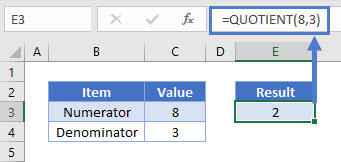The QUOTIENT Function will divide 8 by 3 resulting in 2.66. Here the remainder of division is discarded and the Function returns 2.

## QUOTIENT Function – Decimal Numbers

The QUOTIENT Function can perform division of decimal numbers & returns the integer portion of their division.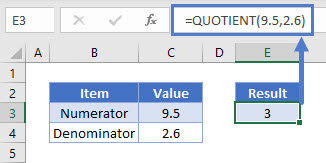## QUOTIENT Function – Negative Numbers

The QUOTIENT Function can perform division of negative numbers & returns the integer portion of their division.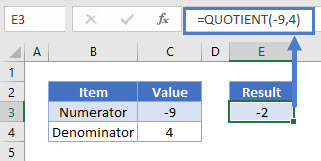## QUOTIENT Function – Divide by 0

The QUOTIENT Function returns an error when the second argument/denominator is zero.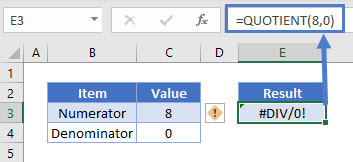## QUOTIENT Function – Error

The QUOTIENT Function returns an error when non-numeric arguments are entered.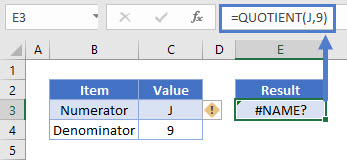## QUOTIENT Function – Finding the remainder of a division

The QUOTIENT Function can be used to find out the remainder of a division.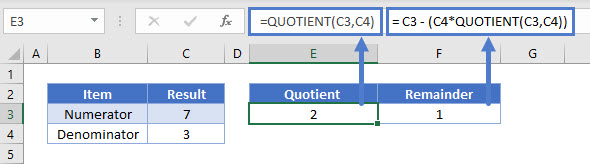Here the formula multiples the divisor/denominator with the quotient (integer portion of the division). It then subtract it from the dividend/numerator to calculate the remainder.

Note: We can also use MOD Function to find out the remainder of a division. To learn more about MOD Function, click here

## QUOTIENT in Google Sheets

The QUOTIENT Function works exactly the same in Google Sheets as in Excel: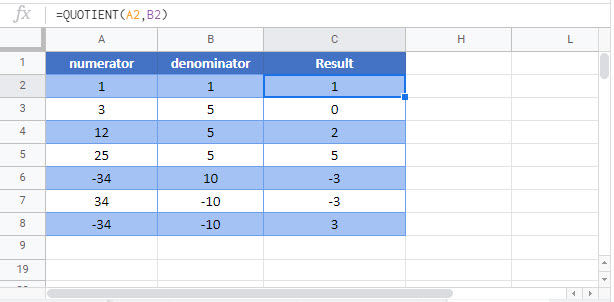## Additional Notes

Use the QUOTIENT Function to calculate the integer result after dividing two numbers.

## QUOTIENT Examples in VBAYou can also use the QUOTIENT function in VBA. Type:
`Application.Worksheetfunction.Quotient(numerator,denominator)`
For the function arguments (numerator, etc.), you can either enter them directly into the function, or define variables to use instead.

Return to the List of all Functions in Excel

## Excel Practice Worksheet

Practice Excel functions and formulas with our 100% free practice worksheets!

• Automatically Graded Exercises
• Learn Excel, Inside Excel!